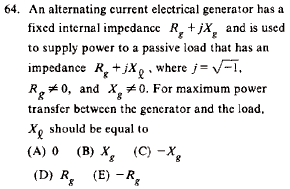GR 8677927796770177 | # Login | Register

GR8677 #64
Problem
 GREPhysics.NET Official Solution Alternate SolutionsElectromagnetism}Impedance Matching

Because the capacitor is fully charged, it is the generator. The inductor, which opposes the current as the capacitor discharges, is the load. Impedance matching requires that the impedance of the generator and load are the same. However, since and , for the generator and load, respectively, and and , one finds that for impedance matching, .Alternate Solutions
 There are no Alternate Solutions for this problem. Be the first to post one!
physics_guy
2018-08-08 21:13:34
There are two kinds of impedance matching. One minimizes reflection at the boundary between materials, and the other maximizes power transfer between two materials. To minimize reflection, the impedance of the generator must equal the impedance of the load. To maximize power transfer, the impedance of the generator must equal the complex conjugate of the impedance of the load. If we wanted to minimize reflection, (B) would be the correct answer. Since we instead want to maximize power transfer, (C) is the correct answer.Marian
2016-01-05 08:57:59
http://http://grephysics.netMarian
2016-01-05 08:56:56
Neige Hunter: montres, nerds pourrait knock réplique Breitling Bentley Flying B plongée en apnée, il est prêt, durée du rythme cardiaque, mais tout le monde peut être dit de se concentrer sur la conception, \"et alors, regardez cette heure génial !.\"\r\n\r\nreplique " target="_blank">http://www.repliquemontresfr.eu\">replique montreMarian
2016-01-05 08:56:09
http://www.repliquemontresfr.eunishamgu
2011-12-19 22:56:11
prove the commutation relation[L_k,r^2]=0 where r is the radius vectorjw111
2008-09-01 15:11:23
You need to know two things
(1) The impedance is the resistance of object in AC circuit.
(2) The imaginary part of impedance control the PHASE of voltage(cross the object) and current (through the object).

In this problem, we can treat the circuit as an IDEA POWER SOURCE + an OBJECT with impedance equal to

with the max power transfer to the OBJECT
the phase of V and I must be inphase that the imaginary part of effective impedance must be .

ThusRebeccaJK42
2007-03-15 14:22:16
Why is it negative Xg and not positive Xg?
 Blake72007-09-21 19:18:17 One needs the 'imaginary' reactances to cancel out and thus 'match the impedance'sharpstones
2006-10-19 13:49:09
What's this about a capacitor and an inductor? Problem 64 from 86 exams says "An alternating current electrical generator has a fixed internal impedance..." ?yosun
2005-12-06 20:24:53
jax: thanks for the correction.jax
2005-12-02 21:27:25
This one confused me at first so let me clarify,

To maximize the power transfer, the impedance needs to be 'matched'. The impedance is 'matched' if the load impedance equals the complex conjugate of the source impedance, .

In our case maximizes the power transfer, so .
 RusFortunat2015-10-21 14:21:02 Now it is clear. Thank youThere are two kinds of impedance matching. One minimizes reflection at the boundary between materials, and the other maximizes power transfer between two materials. To minimize reflection, the impedance of the generator must equal the impedance of the load. To maximize power transfer, the impedance of the generator must equal the complex conjugate of the impedance of the load. If we wanted to minimize reflection, (B) would be the correct answer. Since we instead want to maximize power transfer, (C) is the correct answer.LaTeX syntax supported through dollar sign wrappers $, ex.,$\alpha^2_0$produces . type this... to get...$\int_0^\infty\partial\Rightarrow\ddot{x},\dot{x}\sqrt{z}\langle my \rangle\left( abacadabra \right)_{me}\vec{E}\frac{a}{b}\$